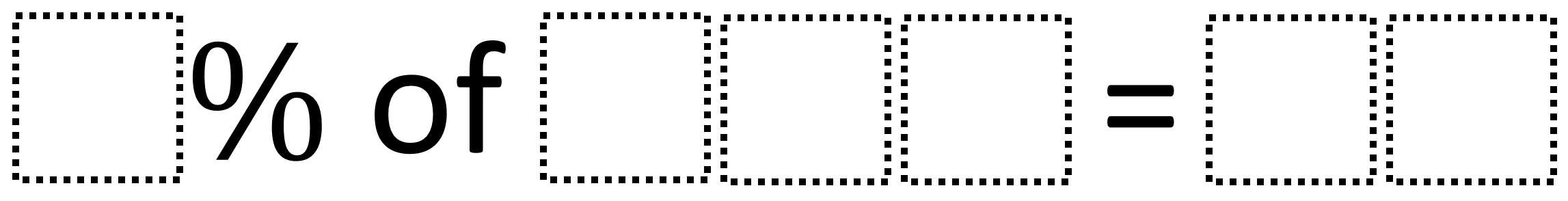# Percentages

Directions: Using the digits 0 to 9 at most one time each, fill in the boxes to create a correct sentence:### Hint

How can we figure out a way to make the two-digit number not have a decimal?

Number of Unique Solutions: 26
1: 2% of 650 = 13
2: 2% of 850 = 17
3: 4% of 925 = 37
4: 4% of 950 = 38
5: 5% of 260 = 13
6: 5% of 280 = 14
7: 5% of 320 = 16
8: 5% of 340 = 17
9: 5% of 360 = 18
10: 5% of 380 = 19
11: 5% of 460 = 23
12: 5% of 620 = 31
13: 5% of 640 = 32
14: 5% of 680 = 34
15: 5% of 720 = 36
16: 5% of 760 = 38
17: 5% of 780 = 39
18: 5% of 820 = 41
19: 5% of 860 = 43
20: 5% of 920 = 46
21: 5% of 960 = 48
22: 6% of 350 = 21
23: 6% of 450 = 27
24: 8% of 450 = 36
25: 8% of 925 = 74
26: 8% of 950 = 76

Source: Cecilia Calvo

## Solving One-Step Inequalities with Addition

Directions: Using the digits 0 to 9 at most one time each, place a digit …

1.Nice! I was going to submit one in a similar vein.

Using the numbers 1-9, at most one time each, fill in the boxes to create a correct sentence:

[] [] % of [] [] is [] []?

68% of 25 is 17
25 % 68 of is 17

25% of 76 is 19
76% of 25 is 19

92% of 75 is 69…

All of the solutions involve a 75 or a 25

Significantly harder:
Three digits!

Using the numbers 0-9, at most one time each, fill in the boxes to create a correct sentence:

[] [] [] % of [] [] []is [] [] [] ?

375 % 184 is 690.

348% of 175 is 609.

2.One answer out of many possible answers is 5% of 260 is 13

3.•I got the same with my program 26 solutions.

4.My student got 8% of 950= 76. Is this a possible highest answer?

•It is indeed!

5.It’s so easy I got 5% of 280=14

6.8% of 59=4.72

7.5% of 260=13 is one of the many solves for this problem

8.I did it this way
9% of 638 = 57

9.6% of 350= 21

10.68% of 50 is 34

11.6% of 350 = 21
6% = 0.06
350 * 0.06 = 21

12.5% of 640 is 32
5% is 0.05
640*0.05 is 32

13.14.2% of 650 = 13